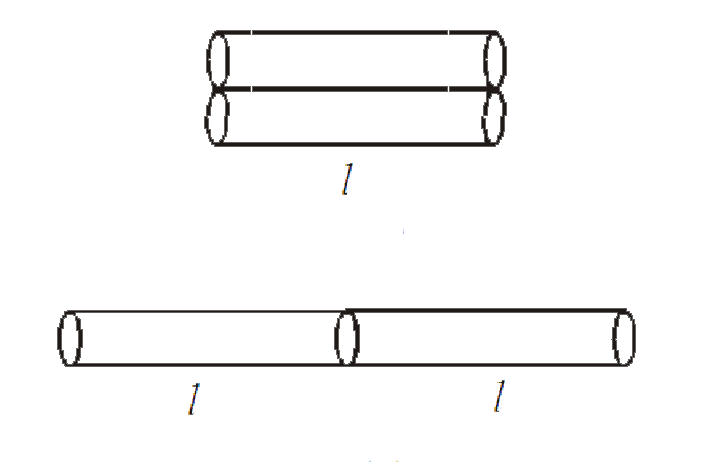# Two rods of same length and transfer a given amount of heat 12 second, when they are joined as shown in figure (i). But when they are joined as shwon

41 views
in Physics
Two rods of same length and transfer a given amount of heat 12 second, when they are joined as shown in figure (i). But when they are joined as shwon in figure (ii), then they will transfer same heat in same conditions inA. 24 s
B. 3 s
C. 1.5 s
D. 48 s

by (76.5k points)